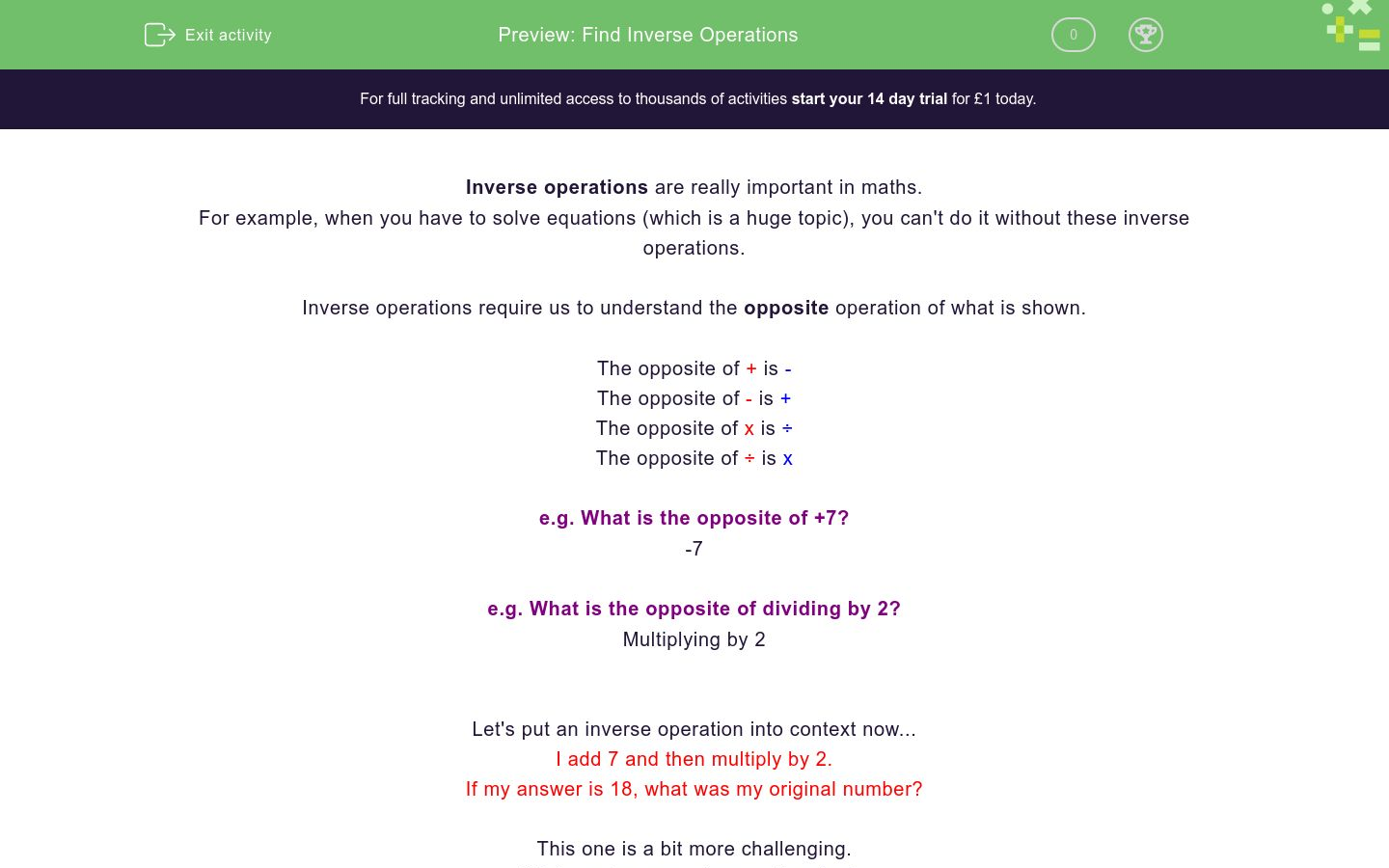Inverse Operations

In this worksheet, students will practise using inverse operations.Key stage:  KS 4

Curriculum topic:

Curriculum subtopic:

Difficulty level:QUESTION 1 of 10

Inverse operations are really important in maths, for example when you have to solve equations (which is a huge topic), you can't do it without these inverse operations.

Inverse operations require us to do the opposite.

The opposite of + is -

The opposite of - is +

The opposite of x is ÷

The opposite of ÷ is x

Examples:

What is the opposite of +7?

Ans: -7

What is the opposite of dividing by 2?

And: Multiplying by 2

I add 7 and then multiply by 2. If my answer is 18, what was my original number?

This one is a bit more challenging. We have two operations, + 7 and x 2. The inverse operations for these would be - 7 and ÷ 2

But which one do we use first?

If we are doing the opposites, we are going backwards. In the question, we added 7 first then multiplied by 2. When we are doing the inverse, we swap this order as well.

Do to solve this we divide by 2 first, then subtract 7.

18 ÷ 2 = 9

9 - 7 = 2

Now it's your turn to have a go!

The inverse of + 4 is...

The inverse operation of ÷ 6 is...

Match the operations with their inverses.

Column B

+ 2
- 2
- 6
+ 6
x 5
x 3
÷ 3
÷ 5

I divide a number by 7 then subtract 4, what are the inverse operations?

Column B

+ 2
- 2
- 6
+ 6
x 5
x 3
÷ 3
÷ 5

I add 7 to a number and get 15. What is the number?

I multiply a number by 6 to get 18. What was my number?

Match the operations with their inverses.

Column B

x 2 + 3
x 2 ÷ 5
+ 3 ÷ 5
-3 ÷ 2
x 5 ÷ 2
x 3 + 7
-7 ÷ 3
x 5 - 3

I subtract 7 from a number and then half it. Which of these is the inverse operation?

x 2 + 7

+ 7 x 2

I double a number and add 6 to get 18. What was my original number?

I half a number and add 4 to get 9. What was my original number?

• Question 1

The inverse of + 4 is...

-4
- 4
EDDIE SAYS
The opposite of + is -, so the opposite of + 4 is...
• Question 2

The inverse operation of ÷ 6 is...

EDDIE SAYS
The opposite of divide is multiply, so the opposite of divide by 6 is... Is this becoming less daunting?
• Question 3

Match the operations with their inverses.

Column B

+ 2
- 2
- 6
+ 6
x 5
÷ 5
÷ 3
x 3
EDDIE SAYS
You just need to remember those inverse operations, + is the opposite of - etc. Once you know the inverse operation, is the number important in this question?
• Question 4

I divide a number by 7 then subtract 4, what are the inverse operations?

EDDIE SAYS
The inverse operation for subtract 4 is add 4. The inverse operation for ÷ 7 is x 7. But did you swap the order? The subtract 4 should have come first.
• Question 5

I add 7 to a number and get 15. What is the number?

8
EDDIE SAYS
The opposite of adding 7 is subtracting 7. If I take 7 away from 15, I get...
• Question 6

I multiply a number by 6 to get 18. What was my number?

3
EDDIE SAYS
The opposite of multiplying by 6 is dividing by 6. If I divide 18 by 6, I get... You're getting better at this with each question!
• Question 7

Match the operations with their inverses.

Column B

x 2 + 3
-3 ÷ 2
+ 3 ÷ 5
x 5 - 3
x 5 ÷ 2
x 2 ÷ 5
-7 ÷ 3
x 3 + 7
EDDIE SAYS
Its all about remembering those inverse operations, + is the opposite of - etc. The key error you could make here is forgetting that we have to swap the order as well!
• Question 8

I subtract 7 from a number and then half it. Which of these is the inverse operation?

x 2 + 7
EDDIE SAYS
The key rule to remember is that when finding the inverse, you must remember to swap the order of operations as well. Great work if you spotted that!
• Question 9

I double a number and add 6 to get 18. What was my original number?

6
EDDIE SAYS
Remember, find the inverses of the operations and then reverse the order. So, (18 - 6) ÷ 2 = ?
• Question 10

I half a number and add 4 to get 9. What was my original number?

10
EDDIE SAYS
Remember, find the inverses of the operations and then reverse the order. So we do, (9-4) x 2 = 10! Well done, that’s another activity completed!
---- OR ----

Sign up for a £1 trial so you can track and measure your child's progress on this activity.

What is EdPlace?

We're your National Curriculum aligned online education content provider helping each child succeed in English, maths and science from year 1 to GCSE. With an EdPlace account you’ll be able to track and measure progress, helping each child achieve their best. We build confidence and attainment by personalising each child’s learning at a level that suits them.

Get startedStart your £1 trial today.
Subscribe from £10/month.